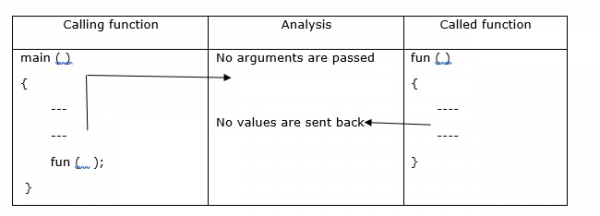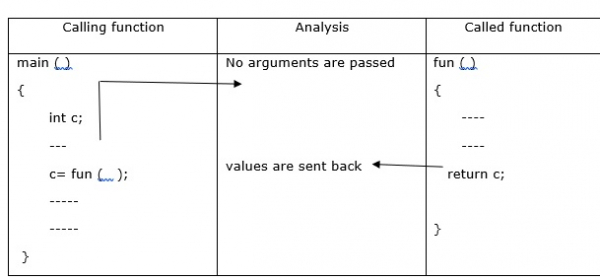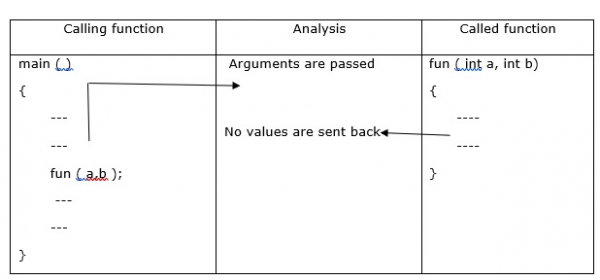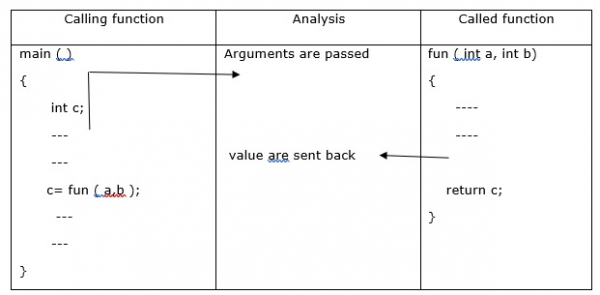# What are the different categories of functions in C Programming?

Depending on whether arguments are present or not and whether a value is returned or not, functions are categorized into −

• Functions without arguments and without return values

• Functions without arguments and with return values

• Functions with arguments and without return values

• Functions with arguments and with return values

## Functions without arguments and without return values## Example

#include<stdio.h>
main (){
void sum ();
clrscr ();
sum ();
getch ();
}
void sum (){
int a,b,c;
printf("enter 2 numbers:");
scanf ("%d%d", &a, &b);
c = a+b;
printf("sum = %d",c);
}

## Output

Enter 2 numbers:
3
5
Sum=8

## Functions without arguments and with return values## Example

#include<stdio.h>
main (){
int sum ();
int c;
c= sum ();
printf(“sum = %d”,c);
getch ();
}
int sum (){
int a,b,c;
printf(“enter 2 numbers”);
scanf (“%d%d”, &a, &b);
c = a+b;
return c;
}

## Output

Enter two numbers 10 20
30

## Functions with arguments and without return values## Example

#include<stdio.h>
main (){
void sum (int, int );
int a,b;
printf("enter 2 numbers");
scanf("%d%d", &a,&b);
sum (a,b);
getch ();
}
void sum ( int a, int b){
int c;
c= a+b;
printf (“sum=%d”, c);
}

## Output

Enter two numbers 10 20
Sum=30

## Functions with arguments and with return values## Example

#include<stdio.h>
main (){
int sum ( int,int);
int a,b,c;
printf("enter 2 numbers");
scanf("%d%d", &a,&b);
c= sum (a,b);
printf ("sum=%d", c);
getch ();
}
int sum ( int a, int b ){
int c;
c= a+b;
return c;
}

## Output

Enter two numbers 10 20
Sum=30